# JP6361769B1 - Position prediction apparatus and position detection apparatus - Google Patents

## Info

Publication number
JP6361769B1
JP6361769B1 JP2017062065A JP2017062065A JP6361769B1 JP 6361769 B1 JP6361769 B1 JP 6361769B1 JP 2017062065 A JP2017062065 A JP 2017062065A JP 2017062065 A JP2017062065 A JP 2017062065A JP 6361769 B1 JP6361769 B1 JP 6361769B1
Authority
JP
Japan
Prior art keywords
position
unit
rotation angle
time
estimated
Prior art date
Legal status (The legal status is an assumption and is not a legal conclusion. Google has not performed a legal analysis and makes no representation as to the accuracy of the status listed.)
Active
Application number
JP2017062065A
Other languages
Japanese (ja)
Other versions
JP2018165619A (en
Inventor

Original Assignee
Tdk株式会社
Ｔｄｋ株式会社
Priority date (The priority date is an assumption and is not a legal conclusion. Google has not performed a legal analysis and makes no representation as to the accuracy of the date listed.)
Filing date
Publication date
Application filed by Tdk株式会社, Ｔｄｋ株式会社 filed Critical Tdk株式会社
Priority to JP2017062065A priority Critical patent/JP6361769B1/en
Application granted granted Critical
Publication of JP6361769B1 publication Critical patent/JP6361769B1/en
Publication of JP2018165619A publication Critical patent/JP2018165619A/en
Application status is Active legal-status Critical

## Images

•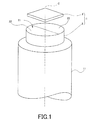•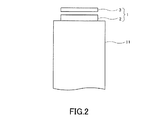•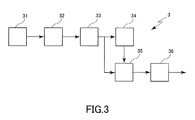•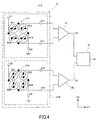•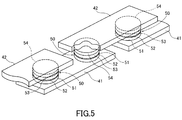•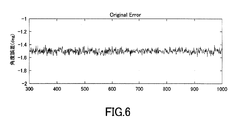•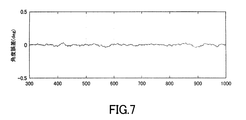•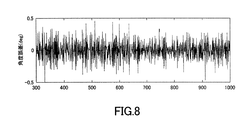•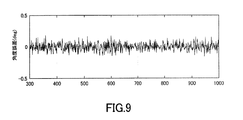••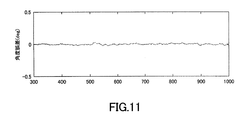•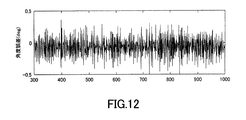•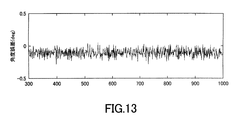•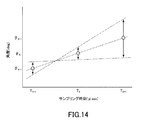## Classifications

• GPHYSICS
• G01MEASURING; TESTING
• G01BMEASURING LENGTH, THICKNESS OR SIMILAR LINEAR DIMENSIONS; MEASURING ANGLES; MEASURING AREAS; MEASURING IRREGULARITIES OF SURFACES OR CONTOURS
• G01B7/00Measuring arrangements characterised by the use of electric or magnetic means
• G01B7/003Measuring arrangements characterised by the use of electric or magnetic means for measuring position, not involving coordinate determination
• GPHYSICS
• G01MEASURING; TESTING
• G01DMEASURING NOT SPECIALLY ADAPTED FOR A SPECIFIC VARIABLE; ARRANGEMENTS FOR MEASURING TWO OR MORE VARIABLES NOT COVERED IN A SINGLE OTHER SUBCLASS; TARIFF METERING APPARATUS; MEASURING OR TESTING NOT OTHERWISE PROVIDED FOR
• G01D5/00Mechanical means for transferring the output of a sensing member; Means for converting the output of a sensing member to another variable where the form or nature of the sensing member does not constrain the means for converting; Transducers not specially adapted for a specific variable
• G01D5/12Mechanical means for transferring the output of a sensing member; Means for converting the output of a sensing member to another variable where the form or nature of the sensing member does not constrain the means for converting; Transducers not specially adapted for a specific variable using electric or magnetic means
• G01D5/14Mechanical means for transferring the output of a sensing member; Means for converting the output of a sensing member to another variable where the form or nature of the sensing member does not constrain the means for converting; Transducers not specially adapted for a specific variable using electric or magnetic means influencing the magnitude of a current or voltage
• G01D5/16Mechanical means for transferring the output of a sensing member; Means for converting the output of a sensing member to another variable where the form or nature of the sensing member does not constrain the means for converting; Transducers not specially adapted for a specific variable using electric or magnetic means influencing the magnitude of a current or voltage by varying resistance
• GPHYSICS
• G01MEASURING; TESTING
• G01BMEASURING LENGTH, THICKNESS OR SIMILAR LINEAR DIMENSIONS; MEASURING ANGLES; MEASURING AREAS; MEASURING IRREGULARITIES OF SURFACES OR CONTOURS
• G01B7/00Measuring arrangements characterised by the use of electric or magnetic means
• G01B7/30Measuring arrangements characterised by the use of electric or magnetic means for measuring angles or tapers; for testing the alignment of axes
• GPHYSICS
• G01MEASURING; TESTING
• G01DMEASURING NOT SPECIALLY ADAPTED FOR A SPECIFIC VARIABLE; ARRANGEMENTS FOR MEASURING TWO OR MORE VARIABLES NOT COVERED IN A SINGLE OTHER SUBCLASS; TARIFF METERING APPARATUS; MEASURING OR TESTING NOT OTHERWISE PROVIDED FOR
• G01D5/00Mechanical means for transferring the output of a sensing member; Means for converting the output of a sensing member to another variable where the form or nature of the sensing member does not constrain the means for converting; Transducers not specially adapted for a specific variable
• G01D5/12Mechanical means for transferring the output of a sensing member; Means for converting the output of a sensing member to another variable where the form or nature of the sensing member does not constrain the means for converting; Transducers not specially adapted for a specific variable using electric or magnetic means
• G01D5/14Mechanical means for transferring the output of a sensing member; Means for converting the output of a sensing member to another variable where the form or nature of the sensing member does not constrain the means for converting; Transducers not specially adapted for a specific variable using electric or magnetic means influencing the magnitude of a current or voltage
• G01D5/142Mechanical means for transferring the output of a sensing member; Means for converting the output of a sensing member to another variable where the form or nature of the sensing member does not constrain the means for converting; Transducers not specially adapted for a specific variable using electric or magnetic means influencing the magnitude of a current or voltage using Hall-effect devices
• G01D5/145Mechanical means for transferring the output of a sensing member; Means for converting the output of a sensing member to another variable where the form or nature of the sensing member does not constrain the means for converting; Transducers not specially adapted for a specific variable using electric or magnetic means influencing the magnitude of a current or voltage using Hall-effect devices influenced by the relative movement between the Hall device and magnetic fields
• GPHYSICS
• G01MEASURING; TESTING
• G01PMEASURING LINEAR OR ANGULAR SPEED, ACCELERATION, DECELERATION, OR SHOCK; INDICATING PRESENCE, ABSENCE, OR DIRECTION, OF MOVEMENT
• G01P3/00Measuring linear or angular speed; Measuring differences of linear or angular speeds
• G01P3/42Devices characterised by the use of electric or magnetic means
• G01P3/44Devices characterised by the use of electric or magnetic means for measuring angular speed
• G01P3/48Devices characterised by the use of electric or magnetic means for measuring angular speed by measuring frequency of generated current or voltage
• G01P3/481Devices characterised by the use of electric or magnetic means for measuring angular speed by measuring frequency of generated current or voltage of pulse signals
• G01P3/487Devices characterised by the use of electric or magnetic means for measuring angular speed by measuring frequency of generated current or voltage of pulse signals delivered by rotating magnets
• GPHYSICS
• G01MEASURING; TESTING
• G01DMEASURING NOT SPECIALLY ADAPTED FOR A SPECIFIC VARIABLE; ARRANGEMENTS FOR MEASURING TWO OR MORE VARIABLES NOT COVERED IN A SINGLE OTHER SUBCLASS; TARIFF METERING APPARATUS; MEASURING OR TESTING NOT OTHERWISE PROVIDED FOR
• G01D5/00Mechanical means for transferring the output of a sensing member; Means for converting the output of a sensing member to another variable where the form or nature of the sensing member does not constrain the means for converting; Transducers not specially adapted for a specific variable
• G01D5/12Mechanical means for transferring the output of a sensing member; Means for converting the output of a sensing member to another variable where the form or nature of the sensing member does not constrain the means for converting; Transducers not specially adapted for a specific variable using electric or magnetic means
• G01D5/244Mechanical means for transferring the output of a sensing member; Means for converting the output of a sensing member to another variable where the form or nature of the sensing member does not constrain the means for converting; Transducers not specially adapted for a specific variable using electric or magnetic means influencing characteristics of pulses or pulse trains; generating pulses or pulse trains
• G01D5/24471Error correction
• G01D5/24495Error correction using previous values

## Abstract

A position predicting device capable of predicting a position of an operating body that operates continuously at a predetermined time with extremely high accuracy and a position detecting device including the position predicting device are provided.
A position prediction apparatus for predicting a position of a moving body that operates continuously at a predetermined time includes an estimation unit that obtains an estimated position state of the moving body at a position estimation time that is earlier than the predetermined time, and an estimation unit that estimates the position. And a position predicting unit that predicts the position of the moving object at a predetermined time based on the estimated position state of the moving object.
[Selection] Figure 1

## Description

The present invention relates to an apparatus for predicting a position due to operation of an operating body and an apparatus for detecting a position due to operation of the operating body.

2. Description of the Related Art Conventionally, a position detecting device that detects the position of an operating body by detecting a change in a physical quantity caused by a rotating operation of an operating body such as a servo motor attached to a machine moving part in a machine tool or the like has been used. By continuously tracking the rotational motion of the operating body through the output from the position detecting device and feeding back to the operating body, the motion control of the operating body is performed.

As such a position detection device, a device including a magnetic field generation unit that generates a magnetic field and a magnetic detection device is known. In general, such a magnetic detection device detects an external magnetic field generated by a magnetic field generation unit and outputs an analog signal indicating a physical quantity to which the magnetic field generation unit has relatively moved, and converts the analog signal into a digital signal. And an arithmetic circuit capable of calculating the position of the operating body at the current time based on the digital signal, and an integrated circuit in which the magnetic detection element and the arithmetic circuit are integrated on the same semiconductor chip. Configured as

Based on the position information of the operating body at the current time calculated by the arithmetic circuit of such a magnetic detection device, motion control of the operating body is performed. However, a filtering process for an analog signal output from the magnetic detection element, a process for converting the analog signal into a digital signal, a filtering process for removing noise contained in the digital signal, and an operating body at the current time based on the digital signal There may be a delay due to the process of calculating the position of. Therefore, in order to accurately control an operating body that operates particularly at high speed, in order to recover the delay, the position of the operating body at a future time is predicted from the position information of the operating body, and the operating body is based on the predicted value. The method of controlling is adopted.

Conventionally, as a position detection device capable of performing such a method, a magnetic sensor element that measures the magnetic field strength of a magnet provided on a rotating body, an angle calculation means that calculates the rotation angle of the magnet from the measurement value of the magnetic sensor element, Storage means for storing rotation angle data output by the angle calculation means, rotation state estimation means for estimating the rotation state by statistically processing the stored contents of the storage means, and the rotation state estimated by the rotation state estimation means A rotation detection device including an extrapolation processing unit that predicts a subsequent rotation angle and an output unit that calculates and outputs the rotation angle based on the rotation angle predicted by the extrapolation processing unit has been proposed (see Patent Document 1). .

JP 2008-116292 A

In the rotation detection device described in Patent Document 1, the angle of the current time is output at a constant sampling period by the magnetic sensor element, and is stored and accumulated in the storage means. Then, the past angle data that has been stored and stored in the storage means and reaches the angle data at the current time is processed by an averaging filter or the like, and the angle (predicted angle) at the sampling time to be predicted is obtained.

The angle data at the current time that is output by the magnetic sensor element at a constant sampling cycle includes predetermined noise. When the angle data including such noise is used to obtain the predicted angle by linear extrapolation processing as in Patent Document 1, there is a problem that the accuracy of the predicted angle decreases. As is apparent from the predictive model shown in FIG. 14, the angle theta X of a predetermined sampling time T X than the angle theta X-1 of the past sampling time T X-1, respectively predetermined noise (Fig. 14 And an arrow indicates a noise width.) When using the angle data to predict the extrapolation angle θ X + 1 at a sampling time T X + 1 that is later than the predetermined time T X by linear extrapolation, The noise included in the predicted angle θ X + 1 is amplified more than the noise included in the angles θ X and θ X−1 .

In addition, if an attempt is made to obtain a predicted angle by means of an average filter process using the angle data, a group delay is caused thereby, and it is necessary to further set the sampling time to be predicted in the future. If the sampling time to be predicted is further set in the future, there is a problem that noise amplification cannot be suppressed and the accuracy of the prediction angle is lowered.

In order to increase the accuracy of the predicted angle, it is conceivable that the angle data output from the magnetic sensor element is processed by a filter circuit or the like to reduce noise included in the angle data. It is possible to reduce noise included in the angle data by processing using a filter circuit or the like. However, since further delay occurs due to processing by the filter circuit or the like, the sampling time to be predicted has to be set in the future. If the sampling time to be predicted is set in the future in this way, the noise reduced by the filter circuit or the like is amplified again and included in the prediction angle, resulting in a decrease in accuracy of the prediction angle as a result. There is.

In view of the above problems, an object of the present invention is to provide a position prediction device capable of predicting the position of a continuously operating operating body at a predetermined time with extremely high accuracy, and a position detection device including the position prediction device. And

In order to solve the above problems, the present invention is an apparatus for predicting a position of a continuously operating operating body at a predetermined time, and an estimated position state of the operating body at a first time before the predetermined time. An estimation unit for obtaining the position, a position prediction unit for predicting the position of the operating body at the predetermined time based on the estimated position state of the operating body estimated by the estimating unit, and a magnetic field generation provided in the operating body An arithmetic processing unit that calculates a position state of the operating body based on a signal related to the position of the operating body that is output by detecting an external magnetic field of the unit, and the first time estimated by the estimating unit based on the estimated positional state of the operation member in a past second time, and a simulation unit for determining the simulated position state in the first time of the operation member, wherein the estimation unit, wherein Based on the simulated position state of the operating body at the first time determined by the emulation unit and the position state of the operating body at the first time calculated by the arithmetic processing unit, the operating body providing position prediction device according to claim Rukoto determined the estimated position state in the first time.

In the position prediction apparatus, it is preferable that the position state of the operating body at the first time is the position state at the latest time among the position states of the operating body calculated by the arithmetic processing unit.

In the prediction apparatus, the moving body is a rotating body that rotates about a predetermined rotation axis, and the estimation unit calculates estimated values of a rotation angle, an angular velocity, and an angular acceleration at the position estimation time of the moving body. It is preferable to obtain the estimated position state.

Further, the present invention provides a comprising: the said position predicting device, is arranged to face the magnetic field generating unit composed provided in the operation member, and a detectable detection unit the position of the operation body Provided is a position detection device. In such a position detection device, the detection unit preferably includes a magnetoresistive element.

ADVANTAGE OF THE INVENTION According to this invention, the position detection apparatus which can predict the position in the predetermined time of the operating body which operate | moves continuously can be provided with very high precision, and the position detection apparatus containing the said position prediction apparatus can be provided.

FIG. 1 is a perspective view showing a schematic configuration of a rotation angle detection device according to an embodiment of the present invention. FIG. 2 is a side view showing a schematic configuration of the rotation angle detection device according to the embodiment of the present invention. FIG. 3 is a block diagram showing a schematic configuration of a magnetic detection device according to an embodiment of the present invention. FIG. 4 is a circuit diagram schematically showing a circuit configuration of the detection unit in one embodiment of the present invention. FIG. 5 is a perspective view showing a schematic configuration of an MR element as a magnetic detection element in one embodiment of the present invention. FIG. 6 is a graph showing noise in the models of Example 1 and Comparative Examples 1-2. FIG. 7 is a graph showing a simulation result in the first embodiment. FIG. 8 is a graph showing simulation results in Comparative Example 1. FIG. 9 is a graph showing a simulation result in Comparative Example 2. FIG. 10 is a graph showing noise in the models of Example 2 and Comparative Examples 3 to 4. FIG. 11 is a graph showing a simulation result in the second embodiment. FIG. 12 is a graph showing a simulation result in Comparative Example 3. FIG. 13 is a graph showing a simulation result in Comparative Example 4. FIG. 14 is a graph for explaining that noise included in a prediction value is amplified in a linear prediction model using angle data including noise.

Embodiments of the present invention will be described in detail with reference to the drawings. FIG. 1 is a perspective view illustrating a schematic configuration of a rotation angle detection device according to the present embodiment, FIG. 2 is a side view illustrating a schematic configuration of the rotation angle detection device according to the present embodiment, and FIG. FIG. 4 is a block diagram illustrating a schematic configuration of a magnetic detection device according to the present embodiment, FIG. 4 is a circuit diagram schematically illustrating a circuit configuration of a detection unit according to the present embodiment, and FIG. 5 illustrates magnetic detection according to the present embodiment. It is a perspective view which shows schematic structure of the MR element as an element.

As shown in FIG. 1 and FIG. 2, the rotation angle detection device 1 according to the present embodiment includes a magnet 2 and a magnetic detection device 3 disposed to face the magnet 2. The magnet 2 is fixed to one end in the axial direction of a shaft portion 11 (for example, a motor shaft such as a servomotor) that rotates continuously around the rotation axis C, and in conjunction with the rotation of the shaft portion 11, It rotates around the rotation axis C.

The magnet 2 has an end face 21 orthogonal to the rotation axis C, and N poles 22 and S poles 23 arranged symmetrically about a virtual plane including the rotation axis C, and a direction orthogonal to the rotation axis C (S It is magnetized in a direction from the pole 23 toward the N pole 22 and in a direction perpendicular to the boundary between the N pole 22 and the S pole 23. The magnet 2 generates a magnetic field based on the magnetization it has.

The magnetic detection device 3 is disposed so as to face the end face 21 of the magnet 2 and detects a magnetic field generated by the magnet 2. The rotation angle detection device 1 according to the present embodiment can detect the rotation angle of the magnet 2, that is, the rotation angle of the shaft portion 11 that rotates and moves based on the output of the magnetic detection device 3.

As illustrated in FIG. 3, the magnetic detection device 3 includes a detection unit 31, an A / D (analog-digital) conversion unit 32, an arithmetic processing unit 33, a simulation unit 34, an estimation unit 35, and a prediction unit 36. The detection part 31 detects the magnetic field of the magnet 2 (refer FIG. 1, FIG. 2). The A / D converter 32 converts the analog signal output from the detector 31 into a digital signal. The arithmetic processing unit 33 performs arithmetic processing on the digital signal digitally converted by the A / D conversion unit 32 to calculate the rotation angle θ. The simulation unit 34 simulates the rotation angle θ S at a predetermined sampling time based on the rotation angle θ E , the angular velocity ω E, and the angular velocity α E estimated by the estimation unit 35. The estimation unit 35 rotates at a predetermined sampling time based on the rotation angle θ S simulated by the simulation unit 34 and the latest rotation angle θ among the rotation angles θ calculated by the arithmetic processing unit 33. An angle θ E , an angular velocity ω E and an angular acceleration α E are estimated. The prediction unit 36 predicts the rotation angle θ P at the current sampling time based on the estimation results (the rotation angle θ E , the angular velocity ω E, and the angular acceleration α E ) by the estimation unit 35. In the present embodiment, the position (for example, the motor shaft of a continuously rotating servo motor, etc.) that operates continuously (at least by the arithmetic processing unit 33, the simulating unit 34, the estimating unit 35, and the predicting unit 36). A position prediction device capable of predicting (rotation position) is configured.

As shown in FIG. 4, the detection unit 31 includes a first detection unit 31A and a second detection unit 31B, and each of the first and second detection units 31A and 31B includes at least one magnetic detection element. Including. The detection unit 31 generates a detection signal (analog signal) relating to an angle (rotation angle) formed by the direction of the magnetic field of the magnet 2 with respect to the predetermined direction at a predetermined sampling period (one sampling period is, for example, about 50 to 100 μsec). ,Output.

Each of the first and second detection units 31A and 31B may include a pair of magnetic detection elements connected in series as at least one magnetic detection element. In this case, each of the first and second detection units 31A and 31B includes a first pair of magnetic detection elements connected in series and a second pair of magnetic detection elements connected in series. It has a Wheatstone bridge circuit.

The Wheatstone bridge circuit 311 included in the first detection unit 31A includes a power supply port V1, a ground port G1, two output ports E11 and E12, and a first pair of magnetic detection elements R11 and R12 connected in series. , And a second pair of magnetic detection elements R13 and R14 connected in series. One end of each of the magnetic detection elements R11 and R13 is connected to the power supply port V1. The other end of the magnetic detection element R11 is connected to one end of the magnetic detection element R12 and the output port E11. The other end of the magnetic detection element R13 is connected to one end of the magnetic detection element R14 and the output port E12. The other ends of the magnetic detection elements R12 and R14 are connected to the ground port G1. A power supply voltage having a predetermined magnitude is applied to the power supply port V1, and the ground port G1 is connected to the ground.

The Wheatstone bridge circuit 312 included in the second detection unit 31B has the same configuration as the Wheatstone bridge circuit 311 of the first detection unit 31A, and includes a power supply port V2, a ground port G2, and two output ports E21 and E22. And a first pair of magnetic detection elements R21, R22 connected in series, and a second pair of magnetic detection elements R23, R24 connected in series. One end of each of the magnetic detection elements R21 and R23 is connected to the power supply port V2. The other end of the magnetic detection element R21 is connected to one end of the magnetic detection element R22 and the output port E21. The other end of the magnetic detection element R23 is connected to one end of the magnetic detection element R24 and the output port E22. The other ends of the magnetic detection elements R22 and R24 are connected to the ground port G2. A power supply voltage having a predetermined magnitude is applied to the power supply port V2, and the ground port G2 is connected to the ground.

In the present embodiment, MR elements such as TMR elements and GMR elements can be used as all the magnetic detection elements R11 to R14 and R21 to R24 included in the Wheatstone bridge circuits 311 and 312, and in particular, TMR elements are used. preferable. The TMR element and the GMR element are a magnetization fixed layer whose magnetization direction is fixed, a free layer whose magnetization direction changes according to the direction of an applied magnetic field, and a nonmagnetic layer disposed between the magnetization fixed layer and the free layer. And having a layer.

Specifically, as shown in FIG. 5, the MR element has a plurality of lower electrodes 41, a plurality of MR films 50, and a plurality of upper electrodes 42. The plurality of lower electrodes 41 are provided on a substrate (not shown). Each lower electrode 41 has an elongated shape. A gap is formed between two lower electrodes 41 adjacent to each other in the longitudinal direction of the lower electrode 41. MR films 50 are provided in the vicinity of both ends in the longitudinal direction on the upper surface of the lower electrode 41. The MR film 50 includes a free layer 51, a nonmagnetic layer 52, a magnetization fixed layer 53, and an antiferromagnetic layer 54, which are sequentially stacked from the lower electrode 41 side. The free layer 51 is electrically connected to the lower electrode 41. The antiferromagnetic layer 54 is made of an antiferromagnetic material and plays a role of fixing the magnetization direction of the magnetization fixed layer 53 by generating exchange coupling with the magnetization fixed layer 53. The plurality of upper electrodes 42 are provided on the plurality of MR films 50. Each upper electrode 42 has an elongated shape, is disposed on two lower electrodes 41 adjacent to each other in the longitudinal direction of the lower electrode 41, and electrically connects the antiferromagnetic layers 54 of the two adjacent MR films 50 to each other. . The MR film 50 may have a configuration in which a free layer 51, a nonmagnetic layer 52, a magnetization fixed layer 53, and an antiferromagnetic layer 54 are stacked in this order from the upper electrode 42 side. The magnetization pinned layer 53 has a laminated ferrimagnetic layer / non-magnetic intermediate layer / ferromagnetic layer ferrimagnetic structure, and a so-called self-pinned pinned layer (Synthetic) in which both ferromagnetic layers are antiferromagnetically coupled. By using a Ferri Pinned layer or an SFP layer, the antiferromagnetic layer 54 may be omitted.

In the TMR element, the nonmagnetic layer 52 is a tunnel barrier layer. In the GMR element, the nonmagnetic layer 52 is a nonmagnetic conductive layer. In the TMR element and the GMR element, the resistance value changes according to the angle formed by the magnetization direction of the free layer 51 with respect to the magnetization direction of the magnetization fixed layer 53, and this angle is 0 ° (the magnetization directions of each other are parallel). The resistance value is minimized at 180 °, and the resistance value is maximized at 180 ° (mutual magnetization directions are antiparallel).

In FIG. 4, the magnetization directions of the magnetization fixed layers of the magnetic detection elements R11 to R14 and R21 to R24 are represented by solid arrows, and the magnetization directions of the free layers are represented by white arrows. In the first detection unit 31A, the magnetization direction of the magnetization fixed layer of the magnetic detection elements R11 and R14 is a direction parallel to the first direction D1, and the magnetization direction of the magnetization fixed layer of the magnetic detection elements R12 and R13 is The magnetization direction of the magnetization fixed layer of the magnetic detection elements R11 and R14 is antiparallel to the magnetization direction. In the first detection unit 31A, the potential difference between the output ports E11 and E12 changes according to the strength of the component in the first direction D1 of the magnetic field of the magnet 2, and the strength of the magnetic field of the magnet 2 in the first direction D1 is changed. A representative signal is output.

In the second detection unit 31B, the magnetization direction of the magnetization fixed layers of the magnetic detection elements R21 and R24 is the second direction D2 (the direction orthogonal to the first direction D1), and the magnetizations of the magnetic detection elements R22 and R23 The magnetization direction of the fixed layer is antiparallel to the magnetization direction of the magnetization fixed layers of the magnetic detection elements R21 and R24. In the second detection unit 31B, the potential difference between the output ports E21 and E22 changes according to the strength of the component in the second direction D2 of the magnetic field of the magnet 2, and the strength of the magnetic field of the magnet 2 in the second direction D2 is changed. A representative signal is output.

The difference detector 37 outputs a signal corresponding to the potential difference between the output ports E11 and E12 to the A / D converter 32 as the first signal S1. The difference detector 38 outputs a signal corresponding to the potential difference between the output ports E21 and E22 to the A / D converter 32 as the second signal S2.

As shown in FIG. 4, the magnetization direction of the magnetization fixed layer of the magnetic detection elements R11 to R14 in the first detection unit 31A, and the magnetization direction of the magnetization fixed layer of the magnetic detection elements R21 to R24 in the second detection unit 31B Are orthogonal to each other. In this case, the waveform of the first signal S1 is a cosine waveform depending on the rotation angle θ, and the waveform of the second signal S2 is a sine waveform depending on the rotation angle θ. In the present embodiment, the phase of the second signal S2 differs from the phase of the first signal S1 by ¼ of the signal period, that is, π / 2 (90 °).

The A / D conversion unit 32 converts the first and second signals (analog signals related to the rotation angle θ) S1 and S2 output from the detection unit 31 at a predetermined sampling period into digital signals, and the digital signals are Input to the arithmetic processing unit 33.

The arithmetic processing unit 33 performs arithmetic processing on the digital signal converted from the analog signal by the A / D conversion unit 32 and calculates the rotation angle θ of the magnet 2. The arithmetic processing unit 33 is configured by, for example, a microcomputer. The rotation angle θ of the magnet 2 calculated by the arithmetic processing unit 33 is stored in a storage unit (not shown) included in the arithmetic processing unit 33.

The rotation angle θ of the magnet 2 can be calculated by, for example, arc tangent calculation represented by the following formula.
θ = atan (S1 / S2)

Note that, within the range of 360 °, the solution of the rotation angle θ in the above formula has two values that differ by 180 °. However, it is possible to determine whether the true value of the rotation angle θ is one of the two solutions in the above expression, by the positive / negative combination of the first signal S1 and the second signal S2. That is, when the first signal S1 is a positive value, the rotation angle θ is greater than 0 ° and smaller than 180 °. When the first signal S1 is a negative value, the rotation angle θ is greater than 180 ° and smaller than 360 °. When the second signal S2 is a positive value, the rotation angle θ is in the range of 0 ° to less than 90 ° and greater than 270 ° to 360 °. When the second signal S2 has a negative value, the rotation angle θ is greater than 90 ° and smaller than 270 °. The arithmetic processing unit 33 calculates the rotation angle θ within the range of 360 ° based on the above formula and the determination of the positive / negative combination of the first signal S1 and the second signal S2.

The simulation unit 34 estimates the magnet 2 at a predetermined sampling time from the rotation angle θ E , angular velocity ω E, and angular acceleration α E of the magnet 2 at the past sampling time estimated by the estimation unit 35 and stored in the storage unit. The rotation angle θ S of is simulated. For example, the simulation unit 34 performs, for example, extrapolation processing on the rotation angle θ E of the magnet 2 at the past sampling time estimated by the estimation unit 35, so that the rotation angle of the magnet 2 at the predetermined sampling time is performed. θ S can be simulated.

The estimation unit 35 reflects the rotation angle θ of the magnet 2 at a predetermined sampling time on the rotation angle θ S of the magnet 2 obtained by the simulating unit 34 so that the rotation angle θ of the magnet 2 at the predetermined sampling time is reflected. In addition to estimating E , angular velocity ω E and angular acceleration α E are estimated.

The prediction unit 36 predicts the rotation angle θ P of the magnet 2 at the current sampling time based on the rotation angle θ E of the magnet 2, the angular velocity ω E, and the angular acceleration α E estimated by the estimation unit 35. For example, the prediction unit 36 calculates the rotation angle θ P of the magnet 2 at the current sampling time by performing extrapolation processing or the like on the rotation angle θ E of the magnet 2 estimated by the estimation unit 35, for example.

In the rotation angle detection apparatus 1 having the above configuration, when the magnet 2 rotates with the rotation of the shaft portion 11, the magnetic field of the magnet 2 changes. In accordance with the change in the magnetic field, the resistance values of the magnetic detection elements R11 to R14 and R21 to R24 of the detection unit 31 change, and the output ports E11, E12, and the second detection unit 31B of the first detection unit 31A and the second detection unit 31B, respectively. In response to the potential difference between E21 and E22, signals S1 and S2 representing the magnetic field strength of the magnet 2 in the first direction D1 and the second direction D2 are output from the difference detectors 37 and 38 at a predetermined sampling period. Then, the first signal S1 and the second signal S2 output from the difference detectors 37 and 38 are output, and are converted into digital signals by the A / D converter 32. Thereafter, the rotation angle θ of the magnet 2 is calculated by the arithmetic processing unit 33.

In the rotation angle detection apparatus 1 according to the present embodiment, filtering processing for an analog signal based on the output from the detection unit 31, processing for conversion to a digital signal by the A / D conversion unit 32, filtering processing for the digital signal, calculation A delay occurs due to arithmetic processing or the like in the processing unit 33. In order to recover this delay, it is important to predict the rotation angle by the prediction unit 36.

For example, in the rotation angle detection device 1 according to the present embodiment, it is assumed that a delay corresponding to three sampling times is caused by various processes. Then, at the current sampling time T n , the calculation processing unit 33 calculates the rotation angle θ n-3 of the magnet 2 at T n-3 three sampling times before the current sampling time T n . On the other hand, the simulation unit 34, for example, the rotation angle θ En-4 and the angular velocity ω En-4 of the magnet 2 estimated at the time T n-4 four sampling times before the current sampling time T n estimated by the estimation unit 35. Based on the angular acceleration α En-4 , the magnet rotation angle θ Sn-3 at T n-3 three sampling times before the current sampling time T n is obtained by simulation. The estimation unit 35 adds the rotation angle θ n-3 of the magnet 2 calculated by the calculation processing unit 33 (latest at the current sampling time T n ) to the rotation angle θ Sn-3 of the magnet 2 simulated by the simulation unit 34. The rotation angle θ En-3 of the magnet 2 at T n-3 before three sampling times is estimated, and the angular velocity ω En-3 and the angular acceleration α En-3 are estimated. . The prediction unit 36 then rotates the rotation angle of the magnet 2 at the current sampling time T n based on the rotation angle θ En-3 , the angular velocity ω En-3, and the angular acceleration α En-3 estimated by the estimation unit 35. Predict θ Pn .

Here, the magnet rotation angle θ calculated by the arithmetic processing unit 33 includes predetermined noise. If an attempt is made to predict the rotation angle θ Pn at the current sampling time T n based on the rotation angle θ including this noise, the noise included in the predicted value of the predicted rotation angle θ Pn is amplified, and accurate prediction is possible. It becomes difficult. In the prediction method (Japanese Patent Application No. 2015-67498) using the angular velocity ω and the angular acceleration α previously proposed by the present inventor, noise included in the predicted rotation angle θ Pn can be reduced. The predicted value of the rotation angle θ Pn may deviate from the rotation angle θ n calculated by the arithmetic processing unit 33. In this prediction method, the operation member to rotation at high speed, very short period of sampling period (for example, the current back from the sampling time T n degree 3 sampling periods below) the rotational motion of the magnet 2 as long as such suppose speed rotational or constant acceleration rotary motion, the angular velocity omega n-3 and the angular acceleration alpha n-3 in the third sampling time before T n-3, the current and the angular velocity omega n and the angular acceleration alpha n at the sampling time T n Are assumed to be substantially the same, the measured value of the rotation angle θ n-3 of the magnet 2 at T n-3 three sampling times before (the rotation angle θ n calculated by the arithmetic processing unit) -3 ) by predicting the rotation angle θ Pn of the magnet 2 at the current sampling time T n based on the angular velocity ω n-3 and the angular acceleration α n-3 calculated from 3 ), the noise included in the rotation angle θ Pn is reduced. Can be reduced Kill. However, the rotation calculated by the current rotation angle theta n-3 are measured values at 3 sampling time before T n-3 which is used to predict the rotation angle theta Pn of the magnet 2 at the sampling time T n (arithmetic processing unit 33 The angle θ n−3 ) is presumed to cause a problem that the rotation angle θ Pn predicted by the prediction unit 36 deviates from the actual measurement value.

In this regard, in the present embodiment, the rotation angle θ En-3 and the angular velocity ω En-3 at T n-3 three sampling times before are used to predict the rotation angle θ Pn of the magnet 2 at the current sampling time T n . In addition, since the angular acceleration α En-3 is an estimated value, the problem that the rotation angle θ Pn predicted by the prediction unit 36 deviates from the rotation angle θ n calculated by the calculation processing unit 33 can be solved. The rotation angle θ Pn of the magnet 2 at the current sampling time T n can be predicted very accurately.

Thus, the rotation angle θ Pn of the magnet 2 predicted extremely accurately by the prediction unit 36 is applied to a drive circuit (not shown) of an operating body including the shaft portion 11 (for example, a servo motor including a motor shaft). Then, the operation control of the operation body is performed. Therefore, the operation control of the operating body can be performed with high accuracy.

As described above, according to the rotation angle detection device 1 according to this embodiment, the prediction unit 36 uses the rotation angle θ En-3 and the angular velocity ω En used to predict the rotation angle θ Pn at the current sampling time T n . -3 and the angular acceleration α En-3 are the rotations simulated by the simulating unit 34 with the rotation angle θ n-3 at the past sampling time calculated by the arithmetic processing unit 33 when the current sampling time T n. Since it is obtained by reflecting the angle θ Sn-3 , the rotation angle θ Pn at the current sampling time T n is extremely accurate based on the rotation angle θ En-3 , the angular velocity ω En-3 and the angular acceleration α En-3. Can be predicted.

Further, according to the rotation angle detection device 1 according to the present embodiment, the rotation angle θ En-3 and the angular velocity ω estimated by the estimation unit 35 in order to predict the rotation angle θ Pn of the magnet 2 at the current sampling time T n . En-3 and angular acceleration α En-3 are obtained simply by using the rotation angle θ Sn-3 simulated by the simulation unit 34 and the latest rotation angle θ n-3 calculated by the arithmetic processing unit 33. Also, an effect that the amount of information (number of samples) necessary for prediction of the rotation angle θ Pn can be reduced can be achieved.

The embodiment described above is described for facilitating understanding of the present invention, and is not described for limiting the present invention. Therefore, each element disclosed in the above embodiment is intended to include all design changes and equivalents belonging to the technical scope of the present invention.

In the above embodiment, the prediction unit 36 has been described by taking an example of predicting the rotation angle θ Pn at the current sampling time T n , but the present invention is not limited to such an aspect, for example, currently it may predict the rotation angle theta Pn + 3 future (e.g. 3 sampling time info T n + 3 than the sampling time T n.

In the above embodiment, the magnet 2 fixed to the shaft portion 11 is used as the magnetic field generating portion. However, the present invention is not limited to such a mode, and for example, at least one set of N pole and S pole. May be configured such that magnets alternately arranged in a ring shape are used as a magnetic field generation unit, and a magnetic detection device is disposed opposite to the outer periphery of the magnet, or a linear scale is used as a magnetic field generation unit. Also good.

In the above-described embodiment, the shaft 2 to which the magnet 2 is fixed rotates around the rotation axis C, so that the magnet 2 rotates relative to the magnetic detection device 3. It is not limited to. For example, the magnet 2 (shaft portion 11) and the magnetic detection device 3 may rotate in opposite directions, or the magnetism 2 (shaft portion 11) does not rotate and the magnetic detection device 3 rotates. It may be a thing.

EXAMPLES Hereinafter, although an Example etc. are given and this invention is demonstrated further in detail, this invention is not limited to the following Example etc. at all.

[Example 1]
With respect to the prediction of the rotation angle θ Pn at the current sampling time T n by the prediction unit 36 in the magnetic detection device 3 having the configuration shown in FIGS. 3 and 4, a simulation is performed using MATLAB and the predicted value of the rotation angle θ Pn is obtained. The included noise was determined. In this simulation, the magnet 2 rotates at a constant speed of 10,000 deg / sec, the sampling period by the detection unit 31 is 50 μsec, and the noise included in the rotation angle θ calculated by the arithmetic processing unit 33 is ± 0.1 deg. (See FIG. 6) It was assumed that a group delay of 3 sampling times (150 μsec) occurred. Further, the simulating unit 34 performs an extrapolation process using the rotation angle θ En-4 , the angular velocity ω En-4 and the angular acceleration En-4 at T n-4 before 4 sampling times, and T n-3 before 3 sampling times. simulates the rotation angle theta Sn-3, the estimation unit 35, the rotation angle theta En-3 by reflecting the rotation angle theta n-3 of 3 sampling time before T n-3 to the rotation angle theta Sn-3 , The angular velocity ω En-3 and the angular acceleration α En-3 are estimated, and the prediction unit 36 performs a rotation angle by linear extrapolation using these estimated values θ En-3 , ω En-3 , α En-3. θ Pn was predicted. The simulation result is shown in FIG.

[Comparative Example 1]
Rotation at the current sampling time T n by performing a linear extrapolation process using the rotation angle θ n-3n-5 at 3 sampling time T n-3 through T n-5 that has been calculated by the arithmetic processing unit 33 except that the that the prediction of the angle theta Pn was determined noise contained in the prediction value of the rotation angle theta Pn in the same manner as in example 1. The simulation result is shown in FIG.

[Comparative Example 2]
4 sampling time T n-3 ~T n-6 rotation angle θ n-3n-6 moving average filter processing by performing with the angular velocity ω n-3n-6 obtained by differentiating once, respectively, in Thus, noise included in the predicted value of the rotation angle θ Pn was obtained in the same manner as in Example 1 except that the rotation angle θ Pn at the current sampling time T n was predicted. The simulation result is shown in FIG.

As is clear from the results of Example 1, Comparative Example 1 and Comparative Example 2 (see FIGS. 7 to 9), the rotation angle θ En-3 , the angular velocity ω En-3 and the angular acceleration α En estimated by the estimation unit 34. Even if a predetermined noise is included in the rotation angle θ, the rotation angle θ Pn at the current sampling time T n can be predicted very accurately without amplifying the noise even if the rotation angle θ includes a predetermined noise. Was confirmed.

[Example 2]
Assuming that the magnet 2 is rotating at a constant acceleration speed of 2 × 10 8 deg / sec 2 , the noise included in the rotation angle θ calculated by the arithmetic processing unit 33 increases as the speed increases as shown in FIG. Except for the increase, the noise included in the predicted value of the rotation angle θ Pn was obtained in the same manner as in Example 1. The results are shown in FIG.

[Comparative Example 3]
Rotation at the current sampling time T n by performing a linear extrapolation process using the rotation angle θ n-3n-5 at 3 sampling time T n-3 through T n-5 that has been calculated by the arithmetic processing unit 33 except that the that the prediction of the angle theta Pn was determined noise contained in the prediction value of the rotation angle theta Pn in the same manner as in example 2. The simulation result is shown in FIG.

[Comparative Example 4]
4. Moving average filter processing using angular accelerations α n-3 to α n-6 obtained by differentiating the rotation angles θ n-3 to θ n- 6 twice at four sampling times T n-3 to T n-6 is performed. Thus, noise included in the predicted value of the rotation angle θ Pn was obtained in the same manner as in Example 2 except that the rotation angle θ Pn at the current sampling time T n was predicted. The simulation result is shown in FIG.

As is clear from the results of Example 2, Comparative Example 3 and Comparative Example 4 (see FIGS. 11 to 13), the rotation angle θ En-3 , the angular velocity ω En-3 and the angular acceleration α En estimated by the estimation unit 34. Even if a predetermined noise is included in the rotation angle θ by the prediction using -3 , the noise is reduced and the predicted value of the rotation angle θ Pn at the current sampling time T n is a true value (calculation processing) It was confirmed that the rotation angle θ n ) calculated by the unit 33 could be predicted very accurately without deviating from the rotation angle θ n ).

1 ... Rotation angle detection device (position detection device)
2 ... Magnet (magnetic field generator)
DESCRIPTION OF SYMBOLS 3 ... Magnetic detection apparatus 31 ... Detection part 33 ... Operation processing part 34 ... Simulation part 35 ... Estimation part 36 ... Prediction part

## Claims (5)

1. An apparatus for predicting a position at a predetermined time of an operating body that operates continuously,
An estimation unit for obtaining an estimated position state of the operating body at a first time that is earlier than the predetermined time;
A position predicting unit that predicts the position of the moving object at the predetermined time based on the estimated position state of the moving object estimated by the estimating unit ;
An arithmetic processing unit that calculates a position state of the operating body based on a signal related to the position of the operating body that is output by detecting an external magnetic field of a magnetic field generating unit provided in the operating body;
A simulation unit that obtains a simulated position state of the moving body at the first time based on an estimated position state of the moving body at a second time that is past the first time, estimated by the estimating unit;
Equipped with a,
The estimation unit includes the simulated position state of the moving body at the first time obtained by the simulating unit, and the position state of the moving body at the first time calculated by the arithmetic processing unit. based on the position predicting device according to claim Rukoto determined the estimated position state in the first time of the operation member.
2. The position state of the operation body in the first time, according to claim 1, characterized in that said position state in the latest time of said position state of the operation member to be calculated by the arithmetic processor Position prediction device.
3. The operating body is a rotating body that rotates about a predetermined rotation axis;
The estimating unit, position prediction device according to claim 1 or 2, characterized in that determining the rotation angle at the position estimation time of the operation member, the estimated value of the angular velocity and angular acceleration as the state the estimated position.
4. The position prediction apparatus according to any one of claims 1 to 3 ,
Wherein is arranged to face the magnetic field generating portion thus provided for the operation member, the position detecting device characterized by comprising a detectable detection unit the position of the operation member.
5. The position detection device according to claim 4 , wherein the detection unit includes a magnetoresistive effect element.
JP2017062065A 2017-03-28 2017-03-28 Position prediction apparatus and position detection apparatus Active JP6361769B1 (en)

## Priority Applications (1)

Application Number Priority Date Filing Date Title
JP2017062065A JP6361769B1 (en) 2017-03-28 2017-03-28 Position prediction apparatus and position detection apparatus

## Applications Claiming Priority (5)

Application Number Priority Date Filing Date Title
JP2017062065A JP6361769B1 (en) 2017-03-28 2017-03-28 Position prediction apparatus and position detection apparatus
US15/805,289 US10352727B2 (en) 2017-03-28 2017-11-07 Position forecasting apparatus and position detection apparatus
DE102017126610.0A DE102017126610A1 (en) 2017-03-28 2017-11-13 Position prediction device and position detection device
CN201711297303.4A CN108662969A (en) 2017-03-28 2017-12-08 Predicted position device and position detecting device
US16/426,095 US20190277662A1 (en) 2017-03-28 2019-05-30 Position forecasting apparatus and position detection apparatus

## Publications (2)

Publication Number Publication Date
JP6361769B1 true JP6361769B1 (en) 2018-07-25
JP2018165619A JP2018165619A (en) 2018-10-25

# Family

## Family Applications (1)

Application Number Title Priority Date Filing Date
JP2017062065A Active JP6361769B1 (en) 2017-03-28 2017-03-28 Position prediction apparatus and position detection apparatus

## Country Status (4)

US (2) US10352727B2 (en)
JP (1) JP6361769B1 (en)
CN (1) CN108662969A (en)
DE (1) DE102017126610A1 (en)

## Citations (5)

* Cited by examiner, † Cited by third party
Publication number Priority date Publication date Assignee Title
JP2008116300A (en) * 2006-11-02 2008-05-22 Ntn Corp Rotation detector and bearing with the rotation detector
JP2010173373A (en) * 2009-01-27 2010-08-12 Mitsubishi Electric Corp Analysis operation assistance program and information processor for artificial satellite
EP2916107A1 (en) * 2014-03-05 2015-09-09 Siemens Aktiengesellschaft Extrapolation of the exact position for a position encoder with phase-modulated raw signal
JP2016186475A (en) * 2015-03-27 2016-10-27 Tdk株式会社 Position prediction device and position detection device
WO2016204205A1 (en) * 2015-06-17 2016-12-22 旭化成エレクトロニクス株式会社 Detection device, rotation angle detection device, detection method, and program

## Family Cites Families (18)

* Cited by examiner, † Cited by third party
Publication number Priority date Publication date Assignee Title
JP3367260B2 (en) 1995-03-24 2003-01-14 三菱電機株式会社 Encoder device and servo motor control device
GB2414300B (en) 2004-02-12 2006-09-20 Weston Aerospace Signal processing method and apparatus
DE102004046803B4 (en) 2004-09-27 2011-08-18 Infineon Technologies AG, 81669 Method for determining the angular position of a rotating object and rotary encoder
JP4689435B2 (en) * 2004-12-16 2011-05-25 アルプス電気株式会社 Angle detection sensor
EP1882159A1 (en) * 2005-05-11 2008-01-30 Toyota Jidosha Kabushiki Kaisha Rotary electric device drive unit including the same
US7456599B2 (en) 2005-05-31 2008-11-25 Rockwell Automation Technologies, Inc. Position feedback device with prediction
JP4925789B2 (en) 2006-11-02 2012-05-09 Ｎｔｎ株式会社 Rotation detection device and bearing with rotation detection device
EP2221588B1 (en) * 2007-11-20 2015-01-14 Sumida Corporation Rotation angle detecting sensor
US8729889B2 (en) * 2008-01-18 2014-05-20 C-Sigma S.R.L. Method and apparatus for magnetic contactless measurement of angular and linear positions
US8096271B2 (en) * 2009-06-01 2012-01-17 GM Global Technology Operations LLC System and method for determining a camshaft position in a variable valve timing engine
JP5096442B2 (en) * 2009-11-17 2012-12-12 株式会社日立製作所 Rotation angle measuring device, motor system and electric power steering system
EP2564084B1 (en) * 2010-04-26 2014-12-31 Schaeffler Technologies GmbH & Co. KG Roller bearing arrangement with an angle sensor
US9098077B2 (en) * 2010-08-25 2015-08-04 Mitsubishi Electric Corporation Trajectory control device
KR101668673B1 (en) * 2012-12-11 2016-10-24 도요타 지도샤（주） Vehicle state detection device
KR101885009B1 (en) * 2013-08-26 2018-08-02 미쓰비시덴키 가부시키가이샤 Angle error correction device and angle error correction method for position detector
JP6210284B2 (en) * 2013-09-18 2017-10-11 株式会社ジェイテクト Rotation angle detector
JP6157300B2 (en) 2013-09-30 2017-07-05 太平洋セメント株式会社 Cement kiln exhaust gas treatment method
JP6191840B2 (en) * 2015-07-31 2017-09-06 Tdk株式会社 Angle sensor correction device, correction method, and angle sensor

## Patent Citations (5)

* Cited by examiner, † Cited by third party
Publication number Priority date Publication date Assignee Title
JP2008116300A (en) * 2006-11-02 2008-05-22 Ntn Corp Rotation detector and bearing with the rotation detector
JP2010173373A (en) * 2009-01-27 2010-08-12 Mitsubishi Electric Corp Analysis operation assistance program and information processor for artificial satellite
EP2916107A1 (en) * 2014-03-05 2015-09-09 Siemens Aktiengesellschaft Extrapolation of the exact position for a position encoder with phase-modulated raw signal
JP2016186475A (en) * 2015-03-27 2016-10-27 Tdk株式会社 Position prediction device and position detection device
WO2016204205A1 (en) * 2015-06-17 2016-12-22 旭化成エレクトロニクス株式会社 Detection device, rotation angle detection device, detection method, and program

## Also Published As

Publication number Publication date
JP2018165619A (en) 2018-10-25
DE102017126610A1 (en) 2018-10-04
US20190277662A1 (en) 2019-09-12
US20180283903A1 (en) 2018-10-04
CN108662969A (en) 2018-10-16
US10352727B2 (en) 2019-07-16

## Similar Documents

Publication Publication Date Title
US10338159B2 (en) Magnetic-field sensor with a back-bias magnet arrangement
JP4513804B2 (en) Magnetic field detector, current detection device using the same, position detection device, and rotation detection device
JP2011002311A (en) Rotation angle detector and rotational speed detector
JP2011525630A (en) Detecting method of rotational position and rotational speed of gear, detecting device of rotational position and rotational speed of gear, and measuring method of wobbling amount of rotating wheel
JP2009058240A (en) Rotation detector
JP5523571B2 (en) Method for evaluating multiple signals from an angle sensor
CN101395450B (en) Magnetic encoder device
CN101995558A (en) Magnetic sensor
JP2002034278A (en) Pole position detector for motor
CN101253389B (en) Rotation angle detection apparatus
DE112008002741T5 (en) Adaptation of GMR sensors in a bridge
EP2333492B1 (en) Rotational angle measurement apparatus
US8659289B2 (en) Rotating field sensor
US9182459B2 (en) Wireless magnetic position sensor
DE102014116844A1 (en) Non-axial magnetic field angle sensors
JP2010197318A (en) Magnetic field detector and measuring instrument
JP5654455B2 (en) Gear speed detection method and gear speed detection device
US20040017186A1 (en) Magnetic circuit for a rotary position sensor
DE102012201348A1 (en) Sensor
US8664943B2 (en) Position detecting apparatus
US8909489B2 (en) Rotating field sensor
US20120249126A1 (en) Circuits and methods for motion detection
JP2009250984A (en) Measurement method of gear rotating speed and gear rotating speed detector
US8604780B2 (en) Rotating field sensor
JP3487452B2 (en) Magnetic detector

## Legal Events

Date Code Title Description
A621 Written request for application examination

Free format text: JAPANESE INTERMEDIATE CODE: A621

Effective date: 20170328

A131 Notification of reasons for refusal

Free format text: JAPANESE INTERMEDIATE CODE: A131

Effective date: 20180306

A521 Written amendment

Free format text: JAPANESE INTERMEDIATE CODE: A523

Effective date: 20180426

TRDD Decision of grant or rejection written
A01 Written decision to grant a patent or to grant a registration (utility model)

Free format text: JAPANESE INTERMEDIATE CODE: A01

Effective date: 20180529

A61 First payment of annual fees (during grant procedure)

Free format text: JAPANESE INTERMEDIATE CODE: A61

Effective date: 20180611

R150 Certificate of patent or registration of utility model

Ref document number: 6361769

Country of ref document: JP

Free format text: JAPANESE INTERMEDIATE CODE: R150